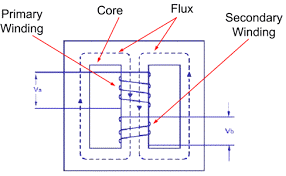Engineering Jobs   »   uprvunl je electrical quiz,

# UPRVUNL’21 EE: Daily Practices Quiz 16-July-2021

Each question carries 1 mark.
Negative marking: 1/4 mark
Total Questions: 06
Time: 08 min.

Q1. Loss of charge method can be used to measure which of the following?
(a) Insulation resistance of cable
(b) Resistance of shunt winding
(c) Resistance of transformer winding
(d) None of the above

Q2. Wien’s bridge is used to measure……….
(a) Energy
(b) Power
(c) Frequency
(d) Phase angle

Q3. In which of the type of transformer primary and secondary winding placed on the same central limb?
(a) Core type transformer
(b) Shell type transformer
(c) Cylindrical type transformer
(d) None of the above

Q4. In synchronous motor damper winding is placed at
(a) Yoke
(b) Stator
(c) Rotor
(d) Both stator and rotor

Q5. Load factor of a power station is generally
(a) Less than unity
(b) Equal to unity
(c) More than unity
(d) Equal to zero

Q6. What is the maximum load that is permitted in a power circuit?
(a) 2000 watts
(b) 3000 watts
(c) 5000 watts
(d) 10000 watts

SOLUTIONS
S1. Ans.(a)
Sol. The loss of charge method is used to measure high values of resistance (insulation resistance).
methods for measurement of high resistance:
Megger
Loss of charge method
Direct deflection method
Megaohm bridge

S2. Ans.(c)
Sol. Wien’s bridge is used to measure frequency.

S3. Ans.(b)
Sol. In shell type of transformer primary and secondary winding are placed on the same central limb.S4. Ans.(c)
Sol. synchronous motors are not self-starting machines. These are made self-starting by providing damper winding on the rotor poles.

S5. Ans.(a)